# ISEE Lower Level Math : Geometry

## Example Questions

### Example Question #1 : How To Find The Points On A Coordinate Plane

Angela plotted points A through E on the coordinate plane shown below.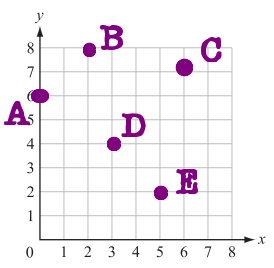What are the coordinates for point B?

(7, 2)

(2, 8)

(8, 8)

(8, 2)

(2, 7)

(2, 8)

Explanation:

Begin at point (0, 0) in the bottom left hand corner of the coordinate plane. From there, count the number of spaces you move to the right to line up with Point B. This will be the first number in the ordered pair. Next, count the number of spaces to move up until you reach Point B. This will be the second number in your ordered pair.

You should have counted 2 spaces to the right and 8 spaces up. The ordered pair for Point B is (2, 8).

### Example Question #1 : How To Find The Points On A Coordinate Plane

Angela plotted points A through E on the coordinate plane shown below.What are the coordinates for Point A?

(1, 6)

(6, 0)

(0, 6)

(0, 5)

(6, 1)

(0, 6)

Explanation:

Begin at point (0, 0) in the bottom left hand corner of the coordinate plane. From there, count the number of spaces you move to the right to line up with Point A. This will be the first number in the ordered pair. Next, count the number of spaces to move up until you reach Point A. This will be the second number in your ordered pair.

You should have counted 0 spaces to the right and 6 spaces up. The ordered pair for Point B is (0, 6).

### Example Question #1 : How To Find The Points On A Coordinate Plane

Angela plotted points A through E on the coordinate plane shown below.Which point is located at the ordered pair (6, 7)?

Point E

Point A

Point B

Point D

Point C

Point C

Explanation:

Start at point (0, 0) in the bottom, left corner of the the coordinate plane. From there, count right 6 spaces. This is the first number in the given ordered pair. From that point, count up 7 spaces. This is the second number in the given ordered pair.

By moving right 6 and up 7, you should have found Point C.

### Example Question #1 : How To Find The Points On A Coordinate Plane

Angela plotted points A through E on the coordinate plane shown below.Which point is located at the ordered pair (2, 8)?

Point E

Point C

Point D

Point A

Point B

Point B

Explanation:

Start at point (0, 0) in the bottom, left corner of the the coordinate plane. From there, count right 2 spaces. This is the first number in the given ordered pair. From that point, count up 8 spaces. This is the second number in the given ordered pair.

By moving right 2 and up 8, you should have found Point B.

### Example Question #1 : How To Find A Parallelogram On A Coordinate Plane

A coordinate plane is shown.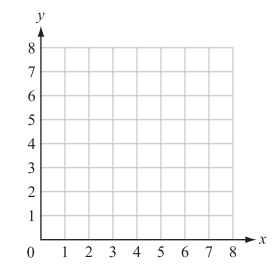Ralph plotted the following points on the coordinate grid:

Point W (2, 4); Point X (3, 6); Point Y (5, 4); Point Z (6, 6)

A polygon is formed with vertices W, X, Y, and Z. Which type of polygon is formed?

Trapezoid

Parallelogram

Square

Kite

Pentagon

Parallelogram

Explanation:

Begin by plotting the points and connecting the vertices.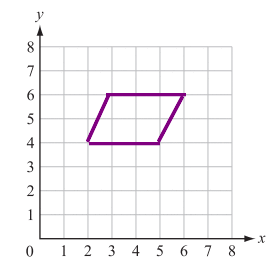The quadrilateral that is created has two sets of parallel sides. Out of the possible answer choices, this can describe both squares and parallelograms. Because the figure does not contain right angles and the sides are not all the same length, it must be a parallelogram.

### Example Question #1 : How To Find A Parallelogram On A Coordinate Plane

A coordinate plane is shown.Ralph plotted the following points on the coordinate grid:

Point W (1, 1); Point X (7, 3); Point Y (1, 6); Point Z (7, 8)

A polygon is formed with vertices W, X, Y, and Z. Which type of polygon is formed?

Hexagon

Triangle

Rhombus

Trapezoid

Parallelogram

Parallelogram

Explanation:

Begin by plotting and connecting the vertices.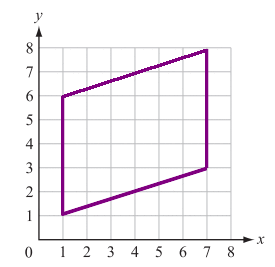The quadrilateral that is created has two sets of parallel sides. Out of the possible answer choices, this can describe both rhombuses and parallelograms. Because the sides are not all the same length, it must be a parallelogram.

### Example Question #11 : Coordinate Geometry

A coordinate plane is shown.Ralph plotted the following points on the coordinate grid:

Point X (7, 0); Point Y (7, 5); Point Z (0, 5)

A polygon is formed with vertices X, Y, and Z. Which type of polygon is formed?

Triangle

Rectangle

Parallelogram

Pentagon

Square

Triangle

Explanation:

Start by graphing and connecting the vertices.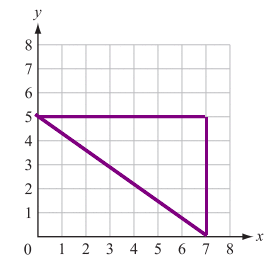The created figure has 3 sides and 3 angles. The only answer choice that has these characteristics is the triangle.

### Example Question #1 : How To Find A Triangle On A Coordinate Plane

A coordinate plane is shown.Ralph plotted the following points on the coordinate grid:

Point X (8, 8); Point Y (1, 4); Point Z (6, 5)

A polygon is formed with vertices X, Y, and Z. Which type of polygon is formed?

Rectangle

Triangle

Square

Triangle

Explanation:

Start by plotting and connecting the ordered pairs.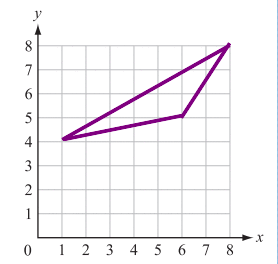The created figure has 3 sides and 3 angles. The only answer choice that has these characteristics is the triangle.

### Example Question #1 : How To Find A Triangle On A Coordinate Plane

Which of the following sets of points would form a triangle if plotted on a coordinate plane?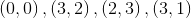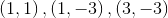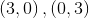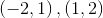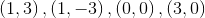Explanation:

A triangle consist of three points.  The correct answer is the only set that contains three points.

### Example Question #1 : How To Find The Perimeter Of A Parallelogram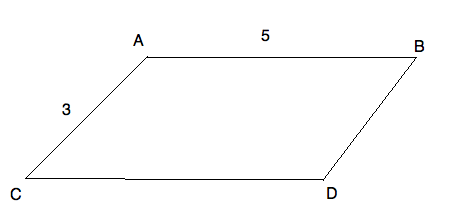What is the perimeter of parallelogram ABCD?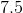Cannot be determinedExplanation:

The perimeter of a parallelogram is very easy to find. You just need to add up all the sides. However, you need to notice that the sides "across" from each other are equal on parallelograms. So, your figure could be redrawn: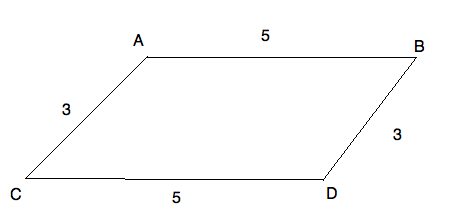The perimeter of your figure is therefore: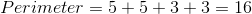### All ISEE Lower Level Math Resources